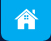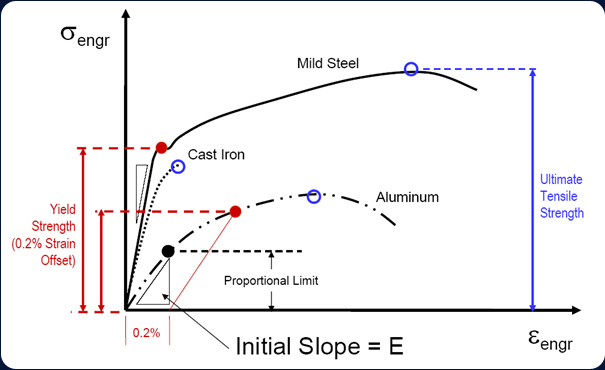# Technical Stress/Strain

FEA (Finite Element Analysis) Simulation use Numerical Method to find approximate Value of Stress & Strain (see *), Validation of this result will be done through Stress Analysis through Strain Gauging Method, This gives Exact Strain Value to set final Design, shall help to Predicts when and where things will break/Fails. Based on that, we can Make design DURABLE and ensure lighter weight, without Compromising Strength that sets Appropriate Safety Factor.

* Stress is the ratio of applied force F and cross section A, defined as "force per area".
* Strain is defined as deformation of a solid due to stress OR Change in length.

### Note:

• Young's Modulus OR Modulus of Elasticity (Hooke's Low) = E. And "E" = Stress / Strain.
• Safety Factor = Ultimate tensile strength / Maximum allowable Stress.

## GRAPH – STRESS Vs STRAIN.

Relationship between STRESS & STRAIN is one of the MOST fundamental Concept derived from the study of materials which are used for STRESS ANALYSIS.